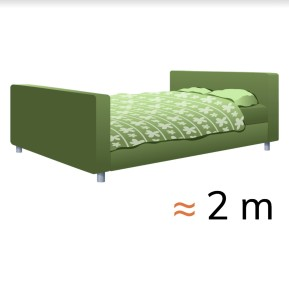Estimating length in meters

# Estimating length in meters8,000 schools use Gynzy92,000 teachers use Gynzy1,600,000 students use Gynzy

## General

Students learn to estimate the length of known objects.

## Common core standard(s)

CCSS.Math.Content.2.MD.A.3

## Learning objective

Students will be able to estimate the length of familiar objects in meters.

## Introduction

Stand in a circle and name an object. Then you throw the ball to a student. They must say whether the object is larger, smaller, or about 1 meter. Next the student comes up with an object and throws the ball to another student, who must give an answer. Repeat this several times.

## Instruction

Explain what a meter is. Show that a blackboard ruler is exactly one meter long. Take a step and say that this is about one meter. Explain that you can estimate the length of objects by comparing them to one big step. This is because you already know that one big step is about one meter. Show this with help from the blackboard ruler. Have the students estimate the size of different objects in the classroom. After students have given an answer, you can measure the objects to check whose estimate is the closest. Next you show images on the interactive whiteboard. The students must stat whether the length of the objects in real life is about three meters or thirty meters. Put the images in the correct box. Afterwards you show other images and the students must estimate how long the object is in real life. They can choose from a few possible answers. You can also name a number of objects yourself, of which the students must estimate the length.

To check whether students can estimate the length of objects in meters, you can ask the following questions:
- What is about one meter?
- How can you estimate the length of an object?

## Quiz

The students first practice with a statement about the length of an object in meters. Then they must estimate the length of the object, for which they can choose from multiple possible answers.

## Closing

You tell the students again that it is useful to be able to estimate the length of objects, so that you know about how long something is. You check whether the students can estimate length in meters by showing several images on the interactive whiteboard. The students must discuss in groups and estimate what the length of the object is in real life. Ask the groups what they have estimated. Afterwards, show the possible answers and have the students discuss again what they think the answer is now. Discuss the answers as a class.

## Teaching tips

When students have difficulty with estimating the length of objects, you can remind them that one big step is about one meter. You can compare objects in real life by taking steps of about one meter. This is how you can estimate the length of an object.

## Instruction materials

A ball and a blackboard ruler.

### The online teaching platform for interactive whiteboards and displays in schools

• Save time building lessons

• Manage the classroom more efficiently

• Increase student engagement